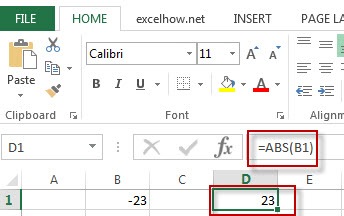# Excel ABS Function

This post will guide you how to use Excel ABS function with syntax and examples in Microsoft excel.

### Description

The Excel ABS function returns the absolute value of a number

The ABS function is a build-in function in Microsoft Excel and it is categorized as a Math and Trigonometry Function.

The ABS function is available in Excel 2016, Excel 2013, Excel 2010, Excel 2007, Excel 2003, Excel XP, Excel 2000, Excel 2011 for Mac.

### Syntax

The syntax of the ABS function is as below:

=ABS (number)

Where the ABS function argument is:
Number – This is a required argument.  A number that you want the absolute value

### Example

The below examples will show you how to use Excel ABS function to return the absolute value of a number.

#1 =ABS(B1)Note: the above formula will return the absolute value of B1 cell.

### More Excel ABS Function Examples

• Sum the Absolute Values
Assuming that you have a list of data in range B1:B6 that contain negative numbers and positive numbers, and you want to sum all absolute values in these range of cells, You can use a formula based on the SUMIF function to achieve the result….